## How to Calculate and Solve for Mass, Volume and Linear Momentum | The Calculator Encyclopedia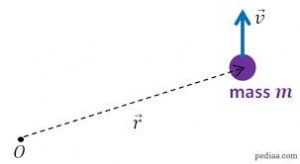The image above represents a linear momentum.

To compute for the linear momentum, two essential parameters are needed and these parameters are mass (m) and velocity (v).

The formula for calculating linear momentum:

p = mv

Where;
p = Momentum
m = Mass
v = Velocity

Let’s solve an example;
Find the linear momentum of a mass of 44 and a velocity of 38.

This implies that;
m = Mass = 44
v = Velocity = 38

p = mv
p = 44 x 38
p = 1672

Therefore, the linear momentum is 1672 Kgm/s.

Calculating the Mass when Linear Momentum and Velocity is Given.

m = p / v

Where;
m = Mass
p = Momentum
v = Velocity

Let’s solve an example;
Find the mass with a linear momentum of 320 and a velocity of 80.

This implies that;
p = Momentum = 320
v = Velocity = 80

m = p / v
m = 320 / 80
m = 4

Therefore, the mass is 4 kg.

## How to Calculate and Solve for Temperature, Number of Moles, Volume, Van’t Hoff Factor and Osmotic Pressure | The Calculator Encyclopedia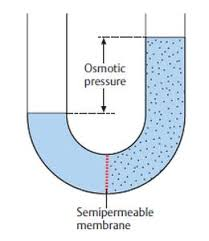The image above represents the osmotic pressure.

To compute for the osmotic pressure, five parameters are needed and these parameters are Ideal Gas Constant (R)Temperature in Kelvin (T), Number of Moles (n), Volume (V) and Van’t Hoff’s Factor (i).

The formula for calculating osmotic pressure:

π = i nRTV

Where;
π = osmotic pressure
n = number of moles
R = ideal gas constant
T = temperature in Kelvin
i = Van’t Hoff’s Factor
V = Volume

Let’s solve an example;
Find the osmotic pressure when the ideal gas constant is 0.08206 with a temperature in kelvin of 120, number of moles is 32, a volume of 48 and a van’t hoff’s factor of 24.

This implies that;
n = number of moles = 32
R = ideal gas constant = 0.08206
T = temperature in Kelvin = 120
i = Van’t Hoff’s Factor = 24
V = Volume = 48

π = i nRTV
π = 24 32 x 0.08206 x 12048
π = (24) (315.110)(48)
π = (24)(6.5647)
π = 157.5

Therefore, the osmotic pressure is 157.5 atm.

Calculating the Van’t Hoff’s Factor using the Osmotic Pressure, Number of Moles, Temperature in Kelvin, Ideal Gas Constant and Volume.

i = / nRT

Where;
i = Van’t Hoff’s Factor
π = osmotic pressure
V = Volume
n = number of moles
R = ideal gas constant
T = temperature in Kelvin

Let’s solve an example;
Find the Van’t Hoff’s Factor when the osmotic pressure is 220, volume of 50, temperature in kelvin of 180 and number of moles of 60. (R = 0.08206)

This implies that;
π = osmotic pressure = 220
V = Volume = 50
n = number of moles = 60
R = ideal gas constant = 0.08206
T = temperature in Kelvin = 180

i = / nRT
i = 50 x 220 / 60 x 0.08206 x 180
i = 11000 / 866.808
i = 12.69

Therefore, the Van’t Hoff’s Factor is 12.69.

Calculating the Volume using the Osmotic Pressure, Number of Moles, Temperature in Kelvin, Ideal Gas Constant and Van’t Hoff’s Factor.

V = i (nRT) / π

Where;
V = Volume
i = Van’t Hoff’s Factor
π = osmotic pressure
n = number of moles
R = ideal gas constant
T = temperature in Kelvin

Let’s solve an example;
Find the volume when the osmotic pressure is 280, Van’t Hoff’s Factor of 40, temperature in kelvin of 90 and number of moles of 70. (R = 0.08206)

This implies that;
i = Van’t Hoff’s Factor = 40
π = osmotic pressure = 280
n = number of moles = 70
R = ideal gas constant = 0.08206
T = temperature in Kelvin = 90

V = i (nRT) / π
V = 40 (70 x 0.08206 x 90) / 280
V = 40 (516.978) / 280
V = 20679.12 / 280
V = 73.854

Therefore, the volume is 73.854.

## How to Calculate and Solve for Mass, Volume and Density | The Calculator Encyclopedia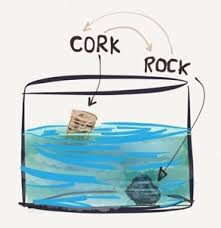The image above represents density.

To compute for the density, two essential parameters are needed and these parameters are mass (m) and volume (v).

The formula for calculating density:

Density = mass / volume

Let’s solve an example;
Given that the volume is 20 m³ with a mass of 240 kg. Find the density?

This implies that;
Volume = 20
Mass = 240

Density = mass / volume
Density = 240 / 20
Density = 12

Therefore, the density is 12 Kg/m³.

Calculating the Mass when the Density and Volume is Given.

Mass = Volume x Density

Let’s solve an example;
With a density of 90 kg/m³ and a volume of 15 m³, Find the mass?

This implies that;
Density = 90
Volume = 15

Mass = Volume x Density
Mass = 15 x 90
Mass = 1350

Therefore, the mass is 1350 kg.

## How to Calculate and Solve for the Mass, Volume and Mass Concentration in Chemistry | The Calculator Encyclopedia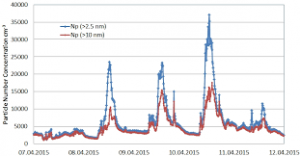The image above represents the mass concentration.

To compute the mass concentration, two essential parameters are needed and these parameters are mass (m) and volume (V).

The formula for calculating mass concentration:

ρ = mV

Where;
ρ = Mass concentration
m = Mass
V = Volume

Let’s solve an example;
Find the mass concentration when the mass is 8 kg with a volume of 24 dm³.

This implies that;
m = Mass = 8
V = Volume = 24

ρ = mV
ρ = 824
ρ = 0.33

Therefore, the mass concentration is 0.33 Kg/dm³.

Calculating the Mass when the Mass Concentration and the Volume is Given.

m = Vρ

Where;
m = Mass
ρ = Mass concentration
V = Volume

Let’s solve an example;
Find the mass when the mass concentration is 12 kg/dm³ with a volume of 7 dm³.

This implies that;
ρ = Mass concentration = 12
V = Volume = 7

m = Vρ
m = 12 x 7
m = 84

Therefore, the mass concentration is 84 kg.

## How to Calculate and Solve for the Number of Moles, Volume and Molar Concentration in Chemistry | Nickzom CalculatorThe image above represents the molar concentration.

To compute the molar concentration of a substance, two essential parameters are needed and these parameters are number of moles (n) and volume (V).

The formula for calculating the molar concentration:

c = nV

Where;
c = Molar Concentration
n = Number of moles
V = Volume

Let’s solve an example;
Find the molar concentration when the number of moles is 32 with a volume of 120.

This implies that;
n = Number of moles = 32
V = Volume = 120

c = nV
c = 32120
c = 0.266

Therefore, the molar concentration is 0.266 mol/L.

Calculating the Number of Moles when Molar Concentration and Volume is Given.

n = Vc

Where;
n = Number of moles
c = Molar Concentration
V = Volume

Let’s solve an example;
Find the number of moles when the molar concentration is 40 with a volume of 31.

This implies that;
c = molar concentration = 40
V = Volume = 31

n = Vc
n = 31 x 40
n = 1240

Therefore, the Number of Moles is 1240 mol.

## How to Calculate and Solve for the Volume of a Constituent, Volume of a Mixture and Volume Concentration in Chemistry | The Calculator EncyclopediaThe image above represents the volume concentration.

To compute the volume concentration of a substance, two essential parameters are needed and these parameters are volume of the constituent (Vi) and volume of the mixture (V).

The formula for calculating volume concentration:

φ = ViV

Where;
φ = Volume concentration
Vi = Volume of the constituent
V = Volume of the mixture

Let’s solve an example;
Find the volume concentration with a volume of the constituent of 23 and a volume of the mixture of 32.

This implies that;
Vi = Volume of the constituent = 23
V = Volume of the mixture = 32

φ = ViV
φ = 2332
φ = 0.718

Therefore, the volume concentration is 0.718.

Calculating the Volume of the Constituent when the Volume Concentration and the Volume of the Mixture is Given.

Vi = Vφ

Where;
φ = Volume concentration
V = Volume of the mixture

Let’s solve an example;
Find the volume of the constituent with a volume concentration of 18 and a volume of the mixture of 22.

This implies that;
φ = Volume concentration = 18
V = Volume of the mixture = 22

Vi = Vφ
Vi = 18 x 22
Vi = 396

Therefore, the volume of the constituent is 396 cm3.

## How to Calculate and Solve for the Number of Entities of a Constituent, Volume and Number Concentration in Chemistry | Nickzom CalculatorThe image above represents the number concentration.

To compute for the number concentration, two essential parameters are needed and these parameters are Number of Entities of the Constituent (N) and Volume (V).

The formula for calculating the number concentration:

C = NV

Where;
C = Number Concentration
N = Number of Entities of the Constituent
V = Volume

Let’s solve an example;
Find the number concentration when the number of entities of the constituent is 12 and the volume is 24.

This implies that;
N = Number of Entities of the Constituent = 12
V = Volume = 24

C = NV
C = 1224
C = 0.5

Therefore, the number concentration is 0.5 m-3.

Calculating the Number of Entities of the Constituent when Number Concentration and Volume is Given.

N = VC

Where;
N = Number of Entities of the Constituent
C = Number Concentration
V = Volume

Let’s solve an example;
Find the number of entities of the constituent when the number concentration is 18 and the volume is 14.

This implies that;
C = Number Concentration = 18
V = Volume = 14

N = VC
N = 14 x 18
N = 252

Therefore, the number of entities of the constituent is 252 m-1.

## How to Calculate and Solve for the Height and Volume of a Conical Frustum | The Calculator Encyclopedia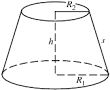The image above is a conical frustum.

To compute the volume of a conical frustum, three essential parameters are needed and this parameters are radius of the lower base (R), radius of the upper base (r) and height (h).

The formula for calculating the volume of a conical frustum:

V = πh(R² + Rr + r²)3

Where;
V = Volume of the conical frustum
R = Radius of the lower base
r = Radius of the upper base
h = Height of the conical frustum

Let’s solve an example;
Find the volume of the conical frustum when the lower base is 5 cm with an upper base of 9 cm and a height of 11 cm.

This implies that;
R = Radius of the lower base = 5 cm
r = Radius of the upper base = 9 cm
h = Height of the conical frustum = 11 cm

V = π(11)((5)² + (5)(9) + (9)²)3
V = (34.557)((25) + (45) + (81))3
V = (34.557)(151)3
V = 5218.1853
V = 1739.39

Therefore, the volume of the conical frustum is 1739.39 cm3.

## How to Calculate and Solve for the Height and Volume of a Square Pyramid Frustum | Nickzom Calculator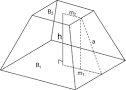The image above is a square pyramid frustum.

To compute the volume of a square pyramid frustum, three essential parameters are needed and this parameters are base side length (a), top side length (b) and height (h).

The formula for calculating the volume of a square pyramid frustum:

V = h(a² + ab + b²)3

Where;
V = Volume of a square pyramid frustum
a = Base side length of the square pyramid frustum
b = Top side length of the square pyramid frustum
h = Height of the square pyramid frustum

Let’s solve an example
Find the volume of a square pyramid frustum when the base side length is 10 cm with a top side length of 12 cm and a height of 20 cm.

This implies that;
a = Base side length of the square pyramid frustum = 10 cm
b = Top side length of the square pyramid frustum = 12 cm
h = Height of the square pyramid frustum = 20 cm

V = h(a² + ab + b²)3
V = 20(10² + (10 x 12) + 12²)3
V = 20(100 + (120) + 144)3
V = 20(364)3
V = 72803
V = 2426.6

Therefore, the volume of the square pyramid frustum is 2426.6 cm3.

Calculating the Height of a Square Pyramid Frustum when the Volume, Top Side Length and Base Side Length of the Square Pyramid Frustum is Given.

h = 3V / (a2 + ab + b2)

Where;
h = Height of the square pyramid frustum
V = Volume of the square pyramid frustum
a = Base side length of the square pyramid frustum
b = Top side length of the square pyramid frustum

Let’s solve an example;
Find the height of a square pyramid frustum when the volume is 32 cm3, base side length is 14 cm and top side length is 18 cm.

This implies that;
V = Volume of the square pyramid frustum = 32 cm3
a = Base side length of the square pyramid frustum = 14 cm
b = Top side length of the square pyramid frustum = 18 cm

h = 3V / (a2 + ab + b2)
h = 32 x 3 / (142 + (14 x 18) + 182)
h = 96 / (196 + 252 + 324)
h = 96 / 772
h = 0.124

Therefore, the height of the square pyramid frustum is 0.124 cm.

## How to Calculate and Solve for the Axis and Volume of an Ellipsoid | The Calculator Encyclopedia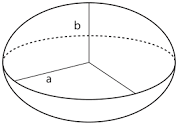The image above is an ellipsoid.

To compute the volume of an ellipsoid, three essential parameters are needed and this parameters are axis (a)axis (b) and axis (c).

The formula for calculating the volume of an ellipsoid:

V = 4πabc3

Where;

V = Volume of the ellipsoid
a = Axis of the ellipsoid
b = Axis of the ellipsoid
c = Axis of the ellipsoid

Let’s solve an example;
Given that the axis of the ellipsoid (a) is 9 cm and axis of the ellipsoid (b) is 11 cm with the axis (c) of 14 cm. Find the volume of the ellipsoid?

This implies that;
a = Axis of the ellipsoid = 9 cm
b = Axis of the ellipsoid = 11 cm
c = Axis of the ellipsoid = 14 cm

V = 4πabc3
V = 4π(9 x 11 x 14)3
V = 4π(1386)3
V = (12.56)(1386) / 3
V = (17416.98967) / 3
V = 5805.66

Therefore, the volume of the ellipsoid is 5805.66 cm3.

Calculating the Axis (a) of an Ellipsoid using the Volume of the Ellipsoid, Axis (b) of the Ellipsoid and Axis (c) of the Ellipsoid.

a = V3 / 4πbc

Where;
a = Axis of the ellipsoid
V = Volume of the ellipsoid
b = Axis of the ellipsoid
c = Axis of the ellipsoid

Let’s solve an example;
Find the axis (a) of an ellipsoid when the volume of the ellipsoid is 280 cm3 with an axis (b) of 18 cm and axis (c) of 8 cm.

This implies that;
V = Volume of the ellipsoid = 280 cm3
b = Axis of the ellipsoid = 18 cm
c = Axis of the ellipsoid = 8 cm

a = V3 / 4πbc
a = 280 x 3 / (12.566)(18 x 8)
a = 840 / (12.566)(144)
a = 840 / 1809.504
a = 0.46

Therefore, the axis (a) of the ellipsoid is 0.46 cm.